# Analyzing Crosstalk Between PCB Traces

This example shows how to create a pair of close traces using `traceLine `object and performs analysis on those traces to calculate the amount of unwanted coupling to the passive trace.

### Create traces

```trace1 = traceLine; trace1.Length = [10 5*sqrt(2) 10 5*sqrt(2) 10]*1e-3; trace1.Angle = [0 45 0 -45 0]; trace1.Width = 3e-3; trace1.Corner = "Miter"; trace2 = copy(trace1); trace2.Length = [11 6*sqrt(2) 6 6*sqrt(2) 11]*1e-3; trace2 = translate(trace2, [0,-5e-3,0]); trace = trace1 + trace2 ; figure; show(trace);```### Create PCB Component

Use the `pcbComponent` to create the PCB stack for the shape. For creating a PCB stack, the trace created above is used as a top layer. The middle layer is a dielectric and the bottom layer is a ground plane. Use the `dielectric `object to create the Teflon dielectric. Use `traceRectangular` object to create a rectangular ground plane. Assign the trace, dielectric(d), and groundplane to the `Layers `property of the `pcbComponent`. Assign the `FeedLocations `at the ends of the trace and visualize it.

```pcb = pcbComponent; d = dielectric('Teflon'); groundplane = traceRectangular('Length', 40e-3,'Width',40e-3,'Center',[40e-3/2,0]); pcb.Layers = {trace,d,groundplane}; pcb.FeedLocations = [0,0,1,3;40e-3,0,1,3;40e-3,-5e-3,1,3;0e-3,-5e-3,1,3]; pcb.BoardShape = groundplane; pcb.FeedDiameter = trace1.Width/2; figure; show(pcb);```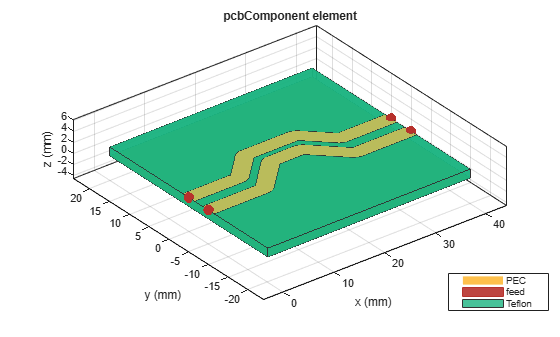Use `current `function to plot the current distribution on the trace

```figure; current(pcb,1e9,'scale','log');```Use the `layout` function to show the layout of the pcbComponent.

```figure; layout(pcb);```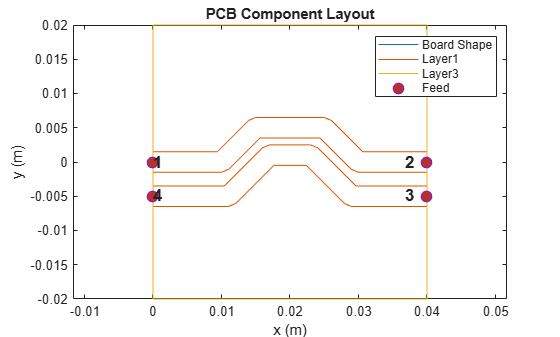Use `sparameters `function to calculate the leakage power from Trace 1 to Trace 2.

```spar = sparameters(pcb,linspace(0.1e9,10e9,51)); figure; rfplot(spar,2:4,1)```The S31 shows the power coupled from the top trace to the bottom trace. This is the unwanted coupling from the top line to the bottom line and must be reduced. To reduce the coupling, increase the spacing between the traces.

### Increase the Spacing Between Traces

```trace1 = traceLine; trace1.Length = [10 5*sqrt(2) 10 5*sqrt(2) 10]*1e-3; trace1.Angle = [0 45 0 -45 0]; trace1.Width = 3e-3; trace1.Corner = "Sharp"; trace2 = copy(trace1); trace2.Length = [11 6*sqrt(2) 6 6*sqrt(2) 11]*1e-3; trace2 = translate(trace2, [0,-10e-3,0]); trace = trace1 + trace2 ; figure; show(trace);```### Create PCB Component

Update the properties of the created earlier and visualize it.

```groundplane = traceRectangular('Length', 40e-3,'Width',40e-3,'Center',[40e-3/2,0]); pcb.Layers = {trace,d,groundplane}; pcb.FeedLocations = [0,0,1,3;40e-3,0,1,3;40e-3,-10e-3,1,3;0e-3,-10e-3,1,3]; figure; show(pcb);```Use `current `function to plot the current distribution on the trace.

```figure; current(pcb,1e9,'scale','log');```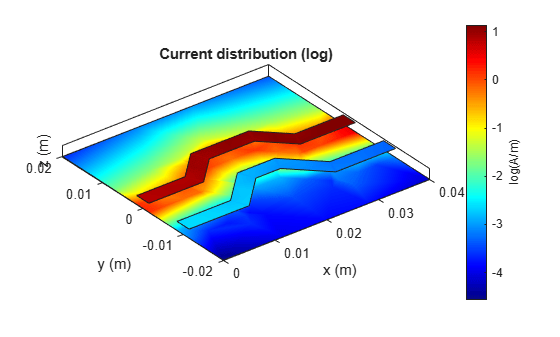Use the `layout` function to show the layout of the `pcbComponent`.

```figure; layout(pcb);```Use `sparameters `function to calculate the leakage power from Trace 1 to Trace 2.

```spar = sparameters(pcb,linspace(0.1e9,10e9,101)); figure; rfplot(spar,2:4,1)```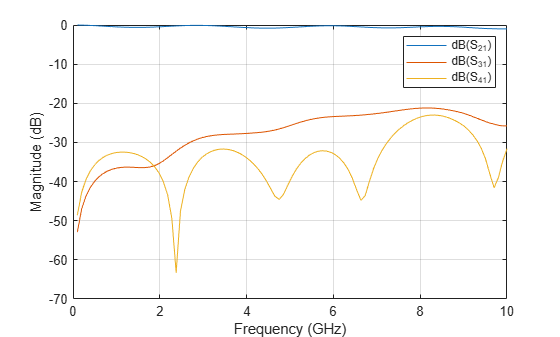When the spacing between the traces is doubled to 10 mm, the coupling also reduces by around 10 dB and reaches to less than -25 dB.

### Plot the Crosstalk Induced Voltage

Define a pulse signal with rise time and fall time and use the `timeresp` function to plot the output signal at port 2 for the input pulse signal.

```sampleTime = 1e-9; t = (0:999)'*sampleTime; input = [0.05*(0:20)';ones(1,78)'; 0.05*(20:-1:0)'; zeros(1,80)']; input = repmat(input,5,1); fit = rationalfit(spar); output = timeresp(fit(3,1),input,sampleTime); figure; yyaxis left; plot(t,input); yyaxis right; plot(t,output); title('Crosstalk between the Traces'); xlabel('Time (sec)'); ylabel('Voltage (volts)'); legend('Vinput','Output','Location','SouthWest');```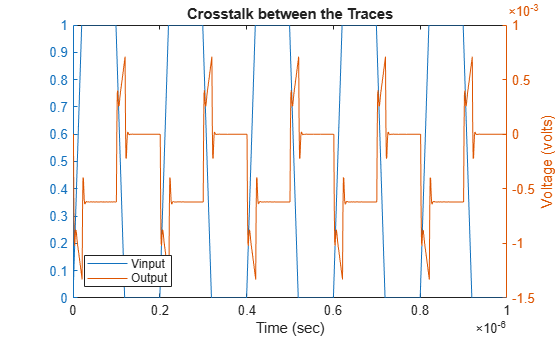The output signal magnitude is around 0.1 mV as seen from the result.

### References

2) Basic Principles of Signal Integrity, Altera